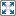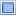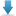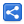# Java Regex - Lookbehind Assertions

[Last Updated: Jan 23, 2016]

Lookbehind is another zero length assertion just like Lookahead assertions. They only assert whether immediate portion behind a given input string's current portion is suitable for a match or not.

There are two kind of lookbehind assertions (just like lookahead): Positive Lookbehind and Negative Lookbehind, and each one of them has two syntax: 'assertion before the match' and 'assertion after the match'. Both of them apply the assertion condition behind their position.

#### Restrictions:

Most of the engines don't support various expressions inside Lookbehind . The reasons is, the engine needs to be able to figure out how many characters to step back before checking the lookbehind expression.
Java allows everything except for '+' and '*' quantifiers(in some cases they work) and backreferences in lookbehind block. In cases like [a-z]*, the said quantifiers work, but they don't work in cases like X[a-z]* (when the expression is bounded on the left)

Note: Unlike Lookbehind, Lookahead assertions support all kind of regex.

Let's start exploring lookbehind with examples.

#### Positive Lookbehind

Positive lookbehind is usually useful if we want to match something preceded by something else.
Syntax: `(?<=X)`

Positive Lookbehind Examples
Positive Lookbehind before the Match

For example: (?<=[0-9])[a-z]

A character preceded by a digit

How engine works?

1. The engine will read the assertion part '(?<=[a-z][0-9])' first.
2. As this assertion is lookbehind, the engine will go behind, in our example by one index less. The engine will see if there's digit at that position. If there's a digit then that satisfies the lookbehind part otherwise it does not.
3. If the last position does not satisfy the lookbehind part, the engine will reject the current position as a match and will move on to the next position.
4. If current position satisfies the lookbehind part then the engine will see what's the next part in the expression which is [a-z]. Now engine will see what is the character at the current position in the input string. If it's a character a to z then it's a match otherwise match will fail and engine will move to the next position.

```Pattern.compile("(?<=[0-9])[a-z]")       .matcher("a9 7c 4t zz")       .find();//matches:  'c' at 4-5, 't' at 7-8
//'a9 7c 4t zz'

/* Note we cannot use unlimited [0-9]+ or [0-9]*, in lookbehind expressions but we can use number range like {3,3} or {3,}*/
Pattern.compile("(?<=[0-9]{3,})[a-z]")       .matcher("a9 447c 455447c 44t zz")       .find();//matches:  'c' at 6-7, 'c' at 14-15
//'a9 447c 455447c 44t zz'

/* Finding alphabets preceded by a digit preceded by an alphabet.*/
Pattern.compile("(?<=[a-z][0-9])[a-z]")       .matcher("64a 5t rr3 a6f")       .find();//matches:  'f' at 13-14
//'64a 5t rr3 a6f'

/* Finding all words of length one or more preceded by a white-space and then a comma.*/
Pattern.compile("(?<=, )[a-z]+")       .matcher("bat, cat, dog, fox")       .find();//matches:  'cat' at 5-8, 'dog' at 10-13, 'fox' at 15-18
//'bat, cat, dog, fox'

/* Finding domain name preceded by 'http://'*/
Pattern.compile("(?<=http://)\\S+")       .matcher("The link is http://www.example.com")       .find();//matches:  'www.example.com' at 19-34

/* Finding all numbers preceded by a white-space preceded by USD*/
Pattern.compile("(?<=USD\\s)\\d+")       .matcher("USD 500, EUR 1000, JPY 50000")       .find();//matches:  '500' at 4-7
//'USD 500, EUR 1000, JPY 50000'

Pattern.compile("(?<=1\\s)[a-z]")       .matcher("1z 1b e4 2c 1 e")       .find();//matches:  'e' at 14-15
//'1z 1b e4 2c 1 e'

/* Finding all text between <h1> and </h1> in an html page. Note that how we used 'positive lookbehind before the match' and 'positive lookahead after the match' together.*/
Pattern.compile("(?<=<h1>)[^<>]+(?=</h1>)")       .matcher("<h1>Regex examples</h1><h1>Another one</h1>")       .find();//matches:  'Regex examples' at 4-18, 'Another one' at 27-38
//'<h1>Regex examples</h1><h1>Another one</h1>'

/* Rewriting above example to use chaining one assertion into another. Here we used positive lookahead 'before the match version' chained inside positive lookbehind 'before the match version'*/
Pattern.compile("(?<=<h1>(?=[^<>]+</h1>))[^<>]+")       .matcher("<h1>Regex examples</h1>")       .find();//matches:  'Regex examples' at 4-18
//'<h1>Regex examples</h1>'

/* Finding english words starting with 'over' but not including 'over' in the match. Note we have to put limit of {0,30} because of we can't use '+' or '*' in the lookbehind assertion if the engine cannot determine the finite length to step back.*/
Pattern.compile("(?<=\\bover[a-z]{0,30})[a-z]*")       .matcher("overall overfill overheat covering")       .find();//matches:  'all' at 4-7, '' at 7-7, 'fill' at 12-16,                //'' at 16-16, 'heat' at 21-25, '' at 25-25
//'overall overfill overheat covering'

```
Positive Lookbehind after the Match

For example [a-z](?<=[0-9][a-z])

A character preceded by a digit.

How engine words?

1. First a character is attempted for a match, satisfying '[a-z]'
2. If the character has matched then engine will read the assertion part '(?<=[0-9])'.
3. As assertion part is of lookbehind the engine will go back by one index position from the current matched position and will see whether at that position the character satisfies the assertion part ( '[0-9]' i.e. if it's a digit). If it is, then the engine will see the next part ('[a-z]' of the assertion) whether it matches the following part of the digit. If it matches too then we have a match otherwise the engine will reject the current character and move to the next position to repeat the process.
4. During reading the assertion part, engine doesn't actually consume any input string for the assertion part, that is it doesn't change the position given by `Matcher#start()` method.

Note that this pattern achieves the same result as last section above ('Positive Lookbehind before the Match'), but it is less efficient because [a-z] is matched twice.

Assertion part [a-z] vs outside part[a-z]:
[a-z](?<=[0-9][a-z])

The two parts can be exactly same. Or assert part [a-z] can be less restrictive (or a subset) as it's applied later than outside part. The concept is same as we saw in Positive Lookahead before the Match discussion.

```Pattern.compile("[a-z](?<=[0-9][a-z])")       .matcher("a9 7c 4t zz")       .find();//matches:  'c' at 4-5, 't' at 7-8
//'a9 7c 4t zz'

/* All alphabet preceded by a digit which in turn preceded by an alphabet.*/
Pattern.compile("[a-z](?<=[a-z][0-9][a-z])")       .matcher("a9 a7 4t zz5t zz")       .find();//matches:  't' at 12-13
//'a9 a7 4t zz5t zz'

/* Finding all numbers having length 1 to 3 having dollar sign before them. Note we cannot use unlimited [0-9]+ inside lookbehind*/
Pattern.compile("\\d+(?<=\\\$[0-9]{1,3})")       .matcher("\$6 \$100 40")       .find();//matches:  '6' at 1-2, '100' at 4-7
//'\$6 \$100 40'

Pattern.compile("[^\\d](?<=.*)")       .matcher("a9 7c 4t zz")       .find();//matches:  'a' at 0-1, ' ' at 2-3, 'c' at 4-5, ' ' at 5-6,                //'t' at 7-8, ' ' at 8-9, 'z' at 9-10, 'z' at 10-11
//'a9 7c 4t zz'

Pattern.compile("[a-z](?<=s)")       .matcher("ages mix uses woes")       .find();//matches:  's' at 3-4, 's' at 10-11, 's' at 12-13, 's' at 17-18
//'ages mix uses woes'

/* This will give us all words ending with a 's'.*/
Pattern.compile("[a-z]+(?<=s)")       .matcher("ages mix uses woes")       .find();//matches:  'ages' at 0-4, 'uses' at 9-13, 'woes' at 14-18
//'ages mix uses woes'

/* Change the quantifier from greedy to reluctant and see the difference.*/
Pattern.compile("[a-z]+?(?<=s)")       .matcher("ages mix uses woes")       .find();//matches:  'ages' at 0-4, 'us' at 9-11, 'es' at 11-13,                //'woes' at 14-18
//'ages mix uses woes'

/* Finding english words containing 'ton' and ending with 'ish'. Notice we have to put a limit {0,} because of the restriction on '*' and '+' with lookbehind expressions.*/
Pattern.compile("[a-z]*ton[a-z]*(?<=[a-z]*ish)")       .matcher("astonish stonefish accomplish")       .find();//matches:  'astonish' at 0-8, 'stonefish' at 9-18
//'astonish stonefish accomplish'

/* Using alteration.*/
Pattern.compile("\\d+ \\w+(?<=\\d+ (thousand|million))")       .matcher("70 million, 12 thousand, 33 hundred")       .find();//matches:  '70 million' at 0-10, '12 thousand' at 12-23
//'70 million, 12 thousand, 33 hundred'

Pattern.compile("\\d(?<=s\\d)")       .matcher("s23e s57e s876e")       .find();//matches:  '2' at 1-2, '5' at 6-7, '8' at 11-12
//'s23e s57e s876e'

Pattern.compile("\\d+(?=e)")       .matcher("ssdfe s57e s876e")       .find();//matches:  '57' at 7-9, '876' at 12-15
//'ssdfe s57e s876e'

```

#### Negative Lookbehind

Negative lookbehind is usually useful if we want to match something not proceeded by something else.

Syntax: `(?<!X)`

Negative Lookbehind Examples
Negative Lookbehind before the Match

For example (?<![0-9])[a-z]
Match an alphabetical character not followed by a digit.

The regex engine works exactly the same as 'Positive Lookbehind before the Match' except that it applies the negation for assertion part.

```/* All characters from a to z not preceded by a digit.*/
Pattern.compile("(?<![0-9])[a-z]")       .matcher("a9 7c 4t zz")       .find();//matches:  'a' at 0-1, 'z' at 9-10, 'z' at 10-11
//'a9 7c 4t zz'

Pattern.compile("(?<![0-9]{3,})[a-z]")       .matcher("a9 447c 455447c 44t zz")       .find();//matches:  'a' at 0-1, 't' at 18-19, 'z' at 20-21, 'z' at 21-22
//'a9 447c 455447c 44t zz'

/* Any alphabet not preceded by b or d or any digits*/
Pattern.compile("(?<!b)(?<!d)(?![0-9])[a-z]")       .matcher("abcde4fgh5ijk")       .find();//matches:  'a' at 0-1, 'b' at 1-2, 'd' at 3-4, 'f' at 6-7,                //'g' at 7-8, 'h' at 8-9, 'i' at 10-11, 'j' at 11-12,                //'k' at 12-13
//'abcde4fgh5ijk'

/* The above assertion is same as this one.*/
Pattern.compile("(?<![ad0-9])[a-z]")       .matcher("abcde4fgh5ijk")       .find();//matches:  'a' at 0-1, 'c' at 2-3, 'd' at 3-4, 'g' at 7-8,                //'h' at 8-9, 'j' at 11-12, 'k' at 12-13
//'abcde4fgh5ijk'

/* The words not preceded by a space '\\s' at word boundary*/
Pattern.compile("(?<!\\s)\\b[a-z]+")       .matcher("food, stand,boss,eyed funny")       .find();//matches:  'food' at 0-4, 'boss' at 12-16, 'eyed' at 17-21
//'food, stand,boss,eyed funny'

/* The words followed by d and word boundary*/
Pattern.compile("\\b[a-z]+(?=d\\b)")       .matcher("food, stand,boss,eyed funny")       .find();//matches:  'foo' at 0-3, 'stan' at 6-10, 'eye' at 17-20
//'food, stand,boss,eyed funny'

/* Combining above two examples: the words not preceded by a white-space at word boundary and ends with d at word boundary.*/
Pattern.compile("(?<!\\s)\\b[a-z]+(?=d\\b)")       .matcher("food, stand,boss,eyed funny")       .find();//matches:  'foo' at 0-3, 'eye' at 17-20
//'food, stand,boss,eyed funny'

/* Rewriting above example to use assertion chaining. But it doesn't give the desired match. That's because the negation of the outer assertion makes the entire block negative.  So preceded by a white-space \\s and ending with d\\b has negation sense as one entity. That's the reason 'funny' is also a one of the undesired match, because it's not satisfying the negation as one entity. In fact it's always a bad idea to apply negation to the outer block while we are chaining. The negation should only be limited to inner block. In next section we will see how can we fix that using 'negative lookbehind after the match'*/
Pattern.compile("(?<!\\s(?=[a-z]+d\\b))\\b[a-z]+")       .matcher("food, stand,boss,eyed, stain, funny")       .find();//matches:  'food' at 0-4, 'boss' at 12-16, 'eyed' at 17-21,                //'stain' at 23-28, 'funny' at 30-35
//'food, stand,boss,eyed, stain, funny'

```
Negative Lookbehind after the Match

For example example [a-z](?<![0-9][a-z])
Match an alphabetical character not preceded by a digit.

The regex engine works exactly the same as 'Positive Lookbehind after the Match' except that it applies the negation for assertion part.

```/* All alphabetical characters not preceded by digits.*/
Pattern.compile("[a-z](?<![0-9][a-z])")       .matcher("a9 7c 4t zz")       .find();//matches:  'a' at 0-1, 'z' at 9-10, 'z' at 10-11
//'a9 7c 4t zz'

/* All digits of max length 3 not preceded by dollar sign.*/
Pattern.compile("\\d+(?<!\\\$[0-9]{1,3})")       .matcher("\$6 \$100 40")       .find();//matches:  '40' at 8-10
//'\$6 \$100 40'

/* All word characters  which are not integers.*/
Pattern.compile("\\w(?<![0-9])")       .matcher("a9 7c 4t zz")       .find();//matches:  'a' at 0-1, 'c' at 4-5, 't' at 7-8, 'z' at 9-10,                //'z' at 10-11
//'a9 7c 4t zz'

/* The words not preceded by a white-space at word boundary and ends with d. Rewriting the example from the last section.*/
Pattern.compile("\\b[a-z]+(?=d\\b(?<!\\s[a-z]{1,30}))")       .matcher("food, stand,boss,eyed funny")       .find();//matches:  'foo' at 0-3, 'eye' at 17-20
//'food, stand,boss,eyed funny'

```

#### Example Project

Dependencies and Technologies Used:

• JDK 1.8
• Maven 3.0.4

 Regex LookbehindSelect AllDownload• regex-lookbehind
• src
• main
• java
• com
• logicbig
• example
• LookBehindExample.java

 Share